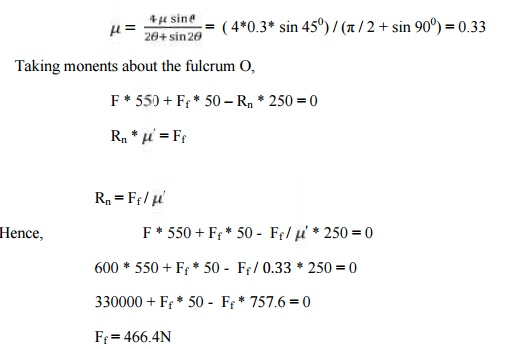Home | | Finite Element Analysis | Solved Problems: Design of Cam Clutches and Brakes

# Solved Problems: Design of Cam Clutches and Brakes

Mechanical - Design of Transmission Systems - Design of Cam Clutches and Brakes

SOLVED PROBLEMs

1.     A single plate clutch with both sides effective; is required to transmit 25 kw at 900 rpm. t hw outer diameter of the plate is 350 mm. the maximum intensity of pressure over the friction surface is not to exceed 0.1 N/mm2 . considering uniform wear criteria and assuming coefficient of friction as 0.25; determine (i) the inner diameter of the plate (ii) axial force required to engage the clutch.

Given;

P= 25 kw ; N =900 rpm ;2r =350 mm ; P max = 0.1 N/mm2 ; µ = 0.25

Sol:-

I.Inner diameter of the plateSubtracting the relation (ii) in (i) and using the values,

r2 [(0.175)2 – r2] = 0.001689

0.031r2 - r2 3 = 0.001689 By trial and error method,

r2 = 0.136m

II.Axial force required to engage the clutch (F)

= 2 π* 0.1 *106 * 0.136* (0.175 – 0.136 ) * 2

= 6665.2 N

2. A multiple  disc clutch transmits 50 kw of power at 1400 rom. Axial intensity of pressure not to exceed 0.12 1 N/mm2, and the coefficient of friction of the friction surfaces is 0.12. the inner radius of the discs is 80 mm, and is .7 times the outer radius. Determine number of disc required to transmit the given power. Assume uniform wear condition.

Given;

P = 60 kw; N = 1400rpm; P max = 0.15 N/mm2; µ = 0.12; r2 = 80mm; r1 = r2/ 0.73. A cone clutch with asbestos friction lining, transmits 25 kw at 600 rpm. The coefficient is 0.25 and the maximum intensity of pressure is 0.25 N/ mm2. The semi cone angle is 12.50. the outer diameter of friction lining is 250 mm . Considering uniform wear theory. Determine

i. the inner diameter of the friction lining,

ii. the face width of friction lining and

iii.the force required to engage to the clutch.

Given;

P = 25kw; N = 600rpm; P max = 0.25 N/mm2; µ = 0.25; α = 12.50; 2r1 = 250mm

Sol;4. A single block brake is shown in figure. The diameter of the drum is 300 mm and the angle of contact is 900. If the operating force of 600N is applied at the end of a lever and the coefficient of friction between the drum and lining is 0.3 determine the torque that may be transmitted by the block brake.

Page no. 27.16(machine desing)

Given;

L = 550mm; x= 250mm; a = 50mm; r = 2θ; 900 = π / 2 rad ; F = 600 N; µ = 0.3

Sol;

In the present case the angle of contact is greater than 600. Hence, the equivalent coefiicient of friction is given by,Torque transmitted by the brake , Tf = Ff * r

= (466.4 * 150 ) / 1000 = 70N-m

Study Material, Lecturing Notes, Assignment, Reference, Wiki description explanation, brief detail
Mechanical : Design of Transmission Systems : Design of Cam Clutches and Brakes : Solved Problems: Design of Cam Clutches and Brakes |

Related Topics